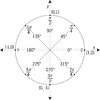# Unit Circle Labeled In 45&deg; Increments With ValuesIllustration of a unit circle (circle with a radius of 1) superimposed on the coordinate plane with the x- and y-axes indicated. At 45° increments, the angles are given in both radian and degree measure. At each quadrantal angle, the coordinates are given. These coordinates can be used to find the six trigonometric values/ratios. The x-coordinate is the value of cosine at the given angle and the y-coordinate is the value of sine. From those ratios, the other 4 trigonometric values can be calculated.

Unit Circles

### Source

Florida Center for Instructional Technology Clipart ETC (Tampa: University of South Florida, 2007)

EPS (vector)

395.9 KiB

TIFF (full resolution)

3000×3000, 219.8 KiB

Large GIF

1024×1024, 33.1 KiB

Medium GIF

640×640, 19.6 KiB

Small GIF

320×320, 8.6 KiB